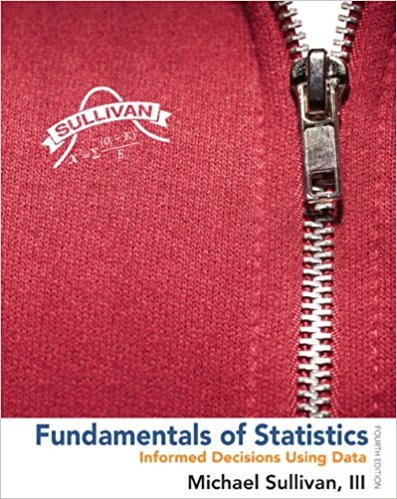×
×

# Solutions for Chapter Chapter 2.2: Fundamentals of Statistics 4th Edition## Full solutions for Fundamentals of Statistics | 4th Edition

ISBN: 9780321838704Solutions for Chapter Chapter 2.2

Solutions for Chapter Chapter 2.2
4 5 0 413 Reviews
13
3
##### ISBN: 9780321838704

Since 68 problems in chapter Chapter 2.2 have been answered, more than 288123 students have viewed full step-by-step solutions from this chapter. This expansive textbook survival guide covers the following chapters and their solutions. This textbook survival guide was created for the textbook: Fundamentals of Statistics, edition: 4. Fundamentals of Statistics was written by and is associated to the ISBN: 9780321838704. Chapter Chapter 2.2 includes 68 full step-by-step solutions.

Key Statistics Terms and definitions covered in this textbook
• Alias

In a fractional factorial experiment when certain factor effects cannot be estimated uniquely, they are said to be aliased.

• Analysis of variance (ANOVA)

A method of decomposing the total variability in a set of observations, as measured by the sum of the squares of these observations from their average, into component sums of squares that are associated with speciic deined sources of variation

• Assignable cause

The portion of the variability in a set of observations that can be traced to speciic causes, such as operators, materials, or equipment. Also called a special cause.

• Bimodal distribution.

A distribution with two modes

• Causal variable

When y fx = ( ) and y is considered to be caused by x, x is sometimes called a causal variable

• Conditional mean

The mean of the conditional probability distribution of a random variable.

• Conditional probability

The probability of an event given that the random experiment produces an outcome in another event.

• Contour plot

A two-dimensional graphic used for a bivariate probability density function that displays curves for which the probability density function is constant.

• Control limits

See Control chart.

• Convolution

A method to derive the probability density function of the sum of two independent random variables from an integral (or sum) of probability density (or mass) functions.

• Decision interval

A parameter in a tabular CUSUM algorithm that is determined from a trade-off between false alarms and the detection of assignable causes.

• Degrees of freedom.

The number of independent comparisons that can be made among the elements of a sample. The term is analogous to the number of degrees of freedom for an object in a dynamic system, which is the number of independent coordinates required to determine the motion of the object.

• Discrete random variable

A random variable with a inite (or countably ininite) range.

• Error variance

The variance of an error term or component in a model.

• Expected value

The expected value of a random variable X is its long-term average or mean value. In the continuous case, the expected value of X is E X xf x dx ( ) = ?? ( ) ? ? where f ( ) x is the density function of the random variable X.

• F distribution.

The distribution of the random variable deined as the ratio of two independent chi-square random variables, each divided by its number of degrees of freedom.

• False alarm

A signal from a control chart when no assignable causes are present

• Frequency distribution

An arrangement of the frequencies of observations in a sample or population according to the values that the observations take on

• Gamma random variable

A random variable that generalizes an Erlang random variable to noninteger values of the parameter r

• Geometric mean.

The geometric mean of a set of n positive data values is the nth root of the product of the data values; that is, g x i n i n = ( ) = / w 1 1 .

×